Open in App
Not now

# C++ Implementation of Scaling in Computer Graphics

• Last Updated : 27 Nov, 2022

In terms of computer graphics, Scaling is a process used for altering the size of objects. It changes the coordinate point of the original object. Modifying the object’s size with the help of the object’s dimension is dependent on the scaling factor(S).

The scaling factor determines whether the object size is increased or decreased. There are two scaling factors i.e., Sx, in the x-direction, and Sy, in the y-direction.

If scaling factor( Sx/Sy) > 1 => size of the object will be enlarged.

If scaling factor( Sx/Sy) < 1 => size of the object will be reduced.

If Sx and Sy are equal then we call it uniform scaling. If both of them are not equal to each other then we can term it as non-uniform scaling or differential scaling or independent scaling. In the non-uniform scaling case, they have the effect of distorting pictures by elongating or shrinking them along the directions parallel to the coordinate axes.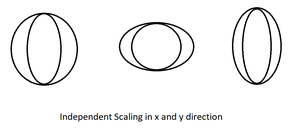Non-uniform scaling factors

### Formula for the Scaling:

Let us have object O on which we will perform the scaling.

initial coordinates of the object O =  (x , y).

scaling factor in x-direction = Sx

scaling factor in y-direction = Sy

new coordinates after scaling = (x1, y1)

hence we have equations for scaling :

• x1 = Sx * x
• y1 = Sy * y

Matrix form of above equations,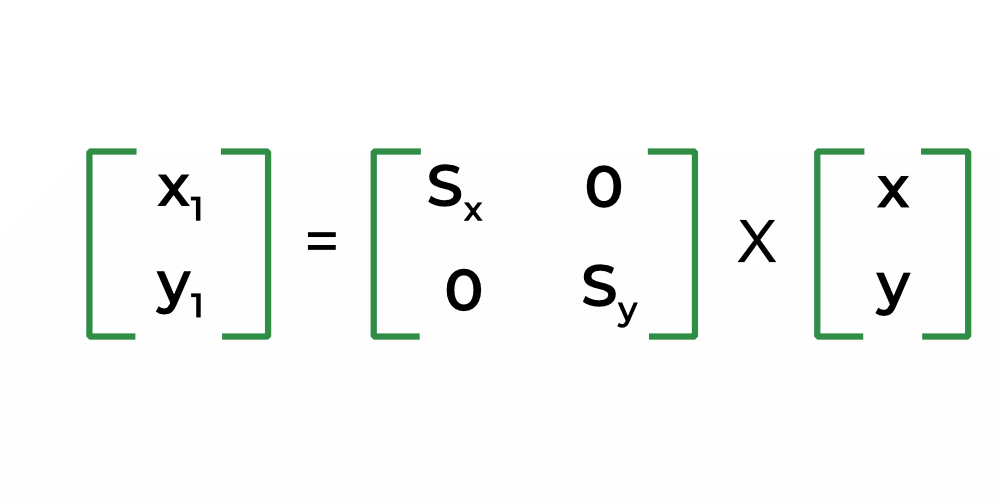matrix representation

For homogeneous coordinates, the above scaling matrix may be represented as a 3X3 matrix,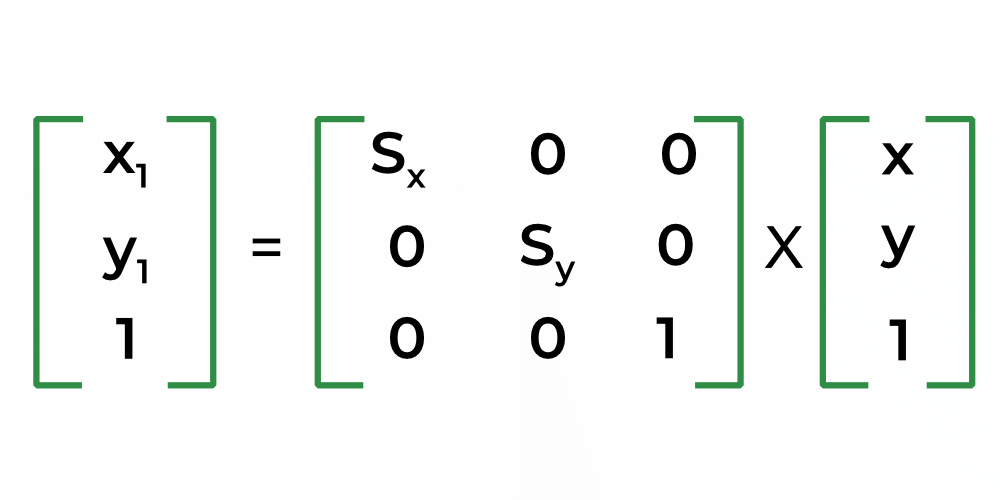Homogeneous representation of scaling equations

For example, we have a triangle with coordinates (20,0), (60,0), and (40, 100) and scaling factors are Sx = Sy = 2. The new coordinates for the object after scaling will be (40, 0), (120, 0), and (80, 200).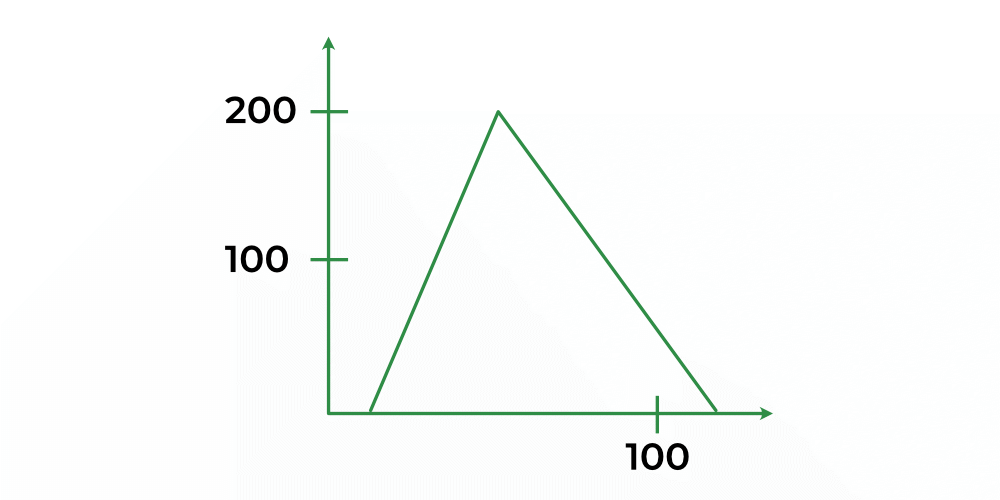Enlarged image of a given example

Here Sx = Sy = 2, indicates the picture of the object to be enlarged to twice its original size. Also, the enlargement is relative to the origin of the coordinate system.

Program For Scaling :

## C++

 `#include ``#include ``#include ``void` `main()``{``    ``int` `gd=DETECT,gm;``    ``float` `p,q,r,s,Sx,Sy;``   ` `    ``initgraph(&gd,&gm,``"C:\\Tc\\BGI"``);`` ` `    ``cout<<``"Enter the first coordinate of a line:"``;``    ``cin>>p>>``" "``>>q;``    ``cout << endl;``   ` `    ``cout<<``"Enter the second coordinate of a line:"``;``    ``cin>>r>>``" "``>>s;``    ``cout << endl;``   ` `    ``line(p,q,r,s);``   ` `    ``cout<<``"Enter the scaling factor:"``;``    ``cin>>Sx>>``" "``>>Sy;``    ``cout << endl;``   ` `    ``p=p*Sx;``    ``q=q*Sy;``    ``r=r*Sx;``    ``s=s*Sy;``   ` `    ``line(p,q,r,s);``   ` `    ``getch();``    ``closegraph();``}`

Output: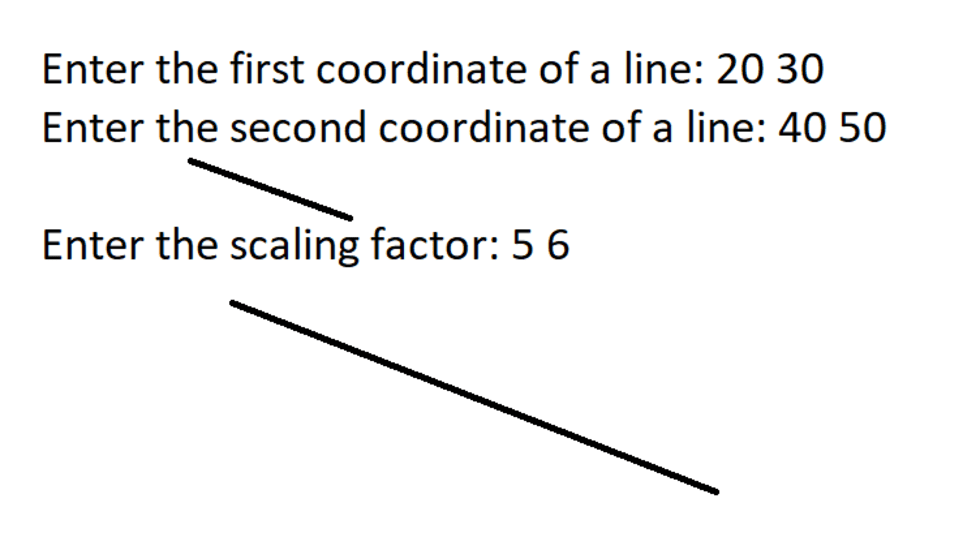the output of the code

My Personal Notes arrow_drop_up